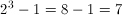PSAT Math : Factors / Multiples

Example Questions

Example Question #5 : How To Factor A Number

The product of three consecutive positive integers is 210. What is the sum of the integers?

6
30
9
21
18
Explanation:

Let x be the first integer. The next two integers would then be x+1 and x+2. We must find x such that:

x(x+1)(x+2) = 210.

To do this, we need to look at the factors of 210, and find a set of three factors that are also consecutive integers. First, let's find the prime factorization of 210, because then we can use that to determine all of the possible factors of 210.

210 = 10 x 21 = 2 x 5 x 21 = 2 x 5 x 3 x 7

Thus, the prime factorization of 210 is 2 x 3 x 5 x 7.

We can notice now that 5 and 7 are almost consecutive integers, and the only number missing between them is 6. If we multiply 2 and 3, we get 6. Thus 210 can be written as 5 x 6 x 7. This means that the consecutive integers are 5, 6, and 7, and their sum is 18.

Example Question #6 : How To Factor A Number

If the variable x is an integer divisible by the numbers 2 and 3, which of the following is necessarily divisible by 2, 3 and 5?

2x + 30

5x + 2

5x + 30

6x + 30

x + 30

5x + 30

Explanation:

For this question, use the fact that a sum of two multiples is a multiple. In other words:

if x is a multiple of 3 and y is a multiple of 3: (x + y) is a multiple of 3.

Thus in this question, x is a multiple of 2 and 3. We need to find a number that is a multiple of 2, 3, and 5.

Take 5x + 30:

[x is divisible by 2. 5 times x is still divisble by 3. 30 is divisible by 2.] -> divisible by 2.

[x is divisible by 3. 5 times x is still divisble by 3. 30 is divisible by 3.] -> divisible by 3.

[5x is divisible by 5. 30 is divisible by 5.] -> divisible by 5.

Thus 5x + 30 is divisible by 2, 3 and 5.

Example Question #1 : How To Factor A Number

If xy = 100 and x and y are distinct positive integers, what is the smallest possible value of xy?

25

20

29

52

101

25

Explanation:

Consider the possible values for (xy):

(1, 100)

(2, 50)

(4, 25)

(5, 20)

Note that (10, 10) is not possible since the two variables are to be distinct. The sums of the above pairs, respectively, are:

1 + 100 = 101

2 + 50 = 52

4 + 25 = 29

5 + 20 = 25, the smallest possible value.

Example Question #8 : How To Factor A Number

If p is an integer, then which of the following could be equal to (6 + 2p)(3)

84

68

75

70

81

84

Explanation:

Let n represent all of the numbers that are equal to (6 + 2p)(3). Then, let's solve for p in terms of n.

(6 + 2p)(3) = n

Divide both sides by 3.

(6 + 2p) = n/3

Subtract six from both sides.

2p = –6 + n/3

Divide both sides by 2.

p = –3 + n/6

Since we are told that p is an integer, the only way that p can be an integer is if n is a multiple of 6. Only a multiple of six, when divided by six, will yield an integer number. Any integer plus –3 will also be an integer, since the sum of two integers is always an integer.

In short, we must look for the answer choice that is a multiple of 6. Of the choices, only 84 is a multiple of 6.

Example Question #1 : How To Factor A Number

What percentage of the positive factors of 48 are also factors of 168?

25

50

100

90

80

80

Explanation:

First, we need to find all of the factors of 48. One way to do this is to come up with all of the pairs of positive integers that will multiply to yield 48. The pairs are as follows:

1 * 48

2 * 24

3 * 16

4 * 12

6 * 8

This means that the facors of 48 are 1, 2, 3, 4, 6, 8, 12, 16, 24, and 48. Note that there are ten factors.

The question asks us for the percentage of the factors of 48 that are also factors of 168. This means we must see which of the ten factors 48 are also factors of 48. One way to do this is do divide 168 by each of the factors of 48 and see which give us whole numbers. If we divide 168 by a factor of 48 and get a whole number, then this number is also a factor of 168.

168/1 = 168

168/2 = 84

168/3 = 56

168/4 = 42

168/6 = 28

168/8 = 21

168/12 = 14

168/16 = 10.5

168/24 = 7

168/48 = 3.5

So, when we divided 168 by the factors of 48, we obtained a whole number, except when we divided by 16 and 48. This means that 16 and 48 are not factors of 168; however, 1, 2, 3, 4, 6, 8, 12, and 24 are all factors of 168.

Thus, out of the ten factors of 48, there are eight which are also factors of 168. We must express 8 out of 10 as a percent. 8/10 = 0.80 = 80%.

Example Question #10 : How To Factor A Number

If there are to be parking spaces at least 6 feet wide along a wall 46 feet long, how many parking space can be drawn in?

5

8

7

4

6

7

Explanation:

Only 7 hole spaces can fit. 8 * 6 = 48, which would be 2 feet longer than the wall.

Example Question #111 : Integers

If k and m are positive integers, let m be equal to the reaminder when k is divided by m. For example, 3 # 2 = 1. All of the following are true EXCEPT:

(k)# k = 0

(km) # k = m

m = 0

0 # (m) = 0

k = m

(km) # k = m

Explanation:

Let's look at each statement separately and see if it must be true.

First, let's consider 0 # (m) = 0.

This statement essentially says that if we divide 0 by the sum of k and m, the remainder should be 0. In general, when 0 is divided by an integer (other than itself), the result is zero with a remainder of zero. For example, if 0 is divided by 2, then 2 will go into zero exactly zero times with a remainder of zero. Because the sum of k and m is an integer, this means that zero divided by (m) will also have a remainder of zero. Thus, 0 # (m) = 0 is true.

Next, let's examine m = 0. This statement says that if we divide m by itself, we should get a remainder of zero. This is true, because any integer divided by itself is equal to 1, with a remainder of zero. Remember that the result of using the # symbol only gives us the remainder. Therefore m # m = 0, not 1.

Let's now look at k # k = m # m.

As we established previously, m # m = 0. Similarly, k = 0, because any integer divided by itself produces a remainder of zero. Because m # m and k # k both equal zero, they are also equal to each other. Thus, k # k = m # m is indeed true.

Next, let's consider (k2) # k = 0. This says that if we square an integer and then divide the result by the integer, we should get a remainder of zero. This is true, because an integer is always a factor of its square. In other words, the square of an integer is always divisible by the integer. And if one number is divisible by another, then the resulting quotient has a remainder of zero. For example (52) # 5 = 0, because when we divide 25 by 5, we get 5 with a remainder of zero. Again, remember that we are only concerned with the remainder.

By the process of elmination, the answer is (km) # k = m. Let's see why this statement must be false. The product of k and m has factors k and m. For example, the product of 4 and 3, which equals 12, has the factors 4 and 3. When a number is divided by one of its factor, the resulting remainder must be zero. For example (4(3)) # 4 = 12 # 4 = 0, because the remainder when 12 is divided by 4 is equal to zero. Thus (km) # k = 0, not m

The answer is (km) # k = m.

Example Question #11 : How To Factor A Number

If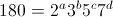, where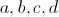are all positive integers, what is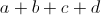?

4

5

7

3

6

5

Explanation:

We will essentially have to represent 180 as a product of prime factors, because 2, 3, 5, and 7 are all prime numbers. The easiest way to do this will be to find the prime factorization of 180.

180 = 18(10)= (9)(2)(10) = (3)(3)(2)(10)=(3)(3)(2)(2)(5) =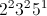. Because 7 is not a factor of 180, we can mutiply the prime factorization of 180 by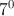(which equals 1) in order to get 7 into our prime factorization.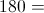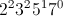=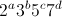In order forto equal, the exponents of each base must match. This means that a = 2, b = 2, c = 1, and d = 0. The sum of a, b, c, and d is 5.

Example Question #62 : Factors / Multiples

What is the product of the distinct prime factors of 24?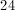Explanation:

The prime factorization of 24 is (2)(2)(2)(3).  The distinct primes are 2 and 3, the product of which is 6.

Example Question #761 : Arithmetic

How many prime factors does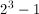have?